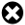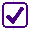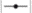# Introduction

Start the activity

In this activity you will:

• explore how a circle can be defined by an equation;
• find out the relationship between the centre and radius of a circle and its equation;
• look at different forms of the equation and learn how to move between the forms yourself.

# How far?

Not Quite!

Not all the points are in the right place.

The green circle that has appeared has radius $5$ units and centre $(2,1)$ . This will help you to think about where $A$, $B$ and $C$ need to be.

Try again, and press the Submit button when they are all correct.

Well Done!

The green circle is all the points that are a distance of $5$ units from $(2,1)$.

Notice that point $B$ is on the circle, point $A$ is inside the circle and point $C$ is outside the circle.

Next you'll think about how to calculate whether a point is on such a circle by looking at its coordinates.

In this activity you need to think about the distance between each green point and the blue point.

Instructions

Position point $A$ so that its distance from $(2,1)$ is less than $5$ units.

Position point $B$ so that it is $5$ units from $(2,1)$.

Position point $C$ so that its distance from $(2,1)$ is greater than $5$ units.

Created with GeoGebra

# Help from Pythagoras

Not Quite!

Think about how Pythagoras's theorem applies to the triangle shown. The hypotenuse of the triangle is the distance between $(x, y)$ and $(2,1)$.

Try again, and press the Submit button when you have done this.

Well Done!

$\sqrt{(x-2)^{2}+(y-1)^{2}}$

is the distance between

$(x, y)$ and $(2,1)$.

To decide whether a point $(x, y)$ is on the circle with radius $5$ centred at $(2,1)$ you need to work out how far $(x, y)$ is from $(2,1)$.

InstructionsTick the expression on the right that gives you the distance between $(x, y)$ and $(2,1)$.

Created with GeoGebra

# Going round in circles

Not Quite!

Try again: read the instructions carefully and look at the coordinates of the green point.

Press the Submit button when you have done this.

Well Done!

$(x-2)^{2}+(y-1)^{2}=25$

is the equation of the circle centred at $(2, 1)$ with radius $5$.

All the points $(x,y)$ on the circle centred at $(2, 1)$ with radius $5$ have the property that:

$\sqrt{(x-2)^{2}+(y-1)^{2}}=5$

This means that, for these points,

$(x-2)^{2}+(y-1)^{2}=25$

Instructions

Move the green point marked $A$. It has been restricted to move only to points $(x,y)$ where

$(x-2)^{2}+(y-1)^{2}=25$.

Watch the calculation of $(x-2)^{2}+(y-1)^{2}$ update as you move the point.

When you have finished exploring, leave the green point in a position with negative $x$ coordinate and negative $y$ coordinate.

Created with GeoGebra

Not Quite!

Try again: look at the centre of the circle and read the instructions carefully. Press the Submit button when you have done this.

Well Done!

The equation of the circle with centre at $(0,0)$ with radius $5$ is:

$x^{2}+y^{2}=25$

Just as the equation of the circle centred at $(2,1)$ with radius $5$ is:

$(x-2)^{2}+(y-1)^{2}=25$,

the equation of the circle with centre at $(a,b)$ with radius $5$ is:

$(x-a)^{2}+(y-b)^{2}=25$

Instructions

Use thesliders to change the values of $a$ and $b$.

Watch carefully how the centre of the circle changes and how the equation of the circle changes.

Leave the circle so that it has centre at $(0,0)$.

Created with GeoGebra

# Stuck in the middle

Not Quite!

Try again, and press the Submit button when you have done this.

Well Done!

You need to take care with minus signs when trying to work out the centre of a circle.

The equation of the circle with radius $5$ and centre $(a,b)$ is

$(x-a)^{2}+(y-b)^{2}=25$

Instructions

Match each of the circle equations on the left to the correct centre on the right by dragging each green point on to the correct blue point.

Created with GeoGebra

Not Quite!

Try again, and press the Submit button when you have done this.

Well Done!

When $r = 0$ the 'circle' becomes a single point.

Here you see the circle with equation:

$(x-2)^{2}+(y-1)^{2}=r^{2}$

Its centre is at $(2, 1)$. Aslider for $r$ is set up with initial value $5$.

Explore how the radius of the circle and the equation of the circle change as you move the slider.

Instructions

Investigate the true/false statements using the slider.Tick those that are true.

Created with GeoGebra

# Different forms...

Not Quite!

Try again, and press the Submit button when you have done this.

Well Done!

So you have two different forms of the equation for the same circle:

$(x-1)^{2}+(y+2)^{2}=9$

and

$x^{2}+y^{2}-2x+4y =4$.

The equation

$(x-2)^{2}+(y-1)^{2}=25$

expands to give:

$x^{2}-4x+4+y^{2}-2y+1=25$

which can then be written as:

$x^{2}-4x+y^{2}-2y=20$

You're now going to explore the relationship between different forms like this.

Instructions

Two curves are shown on the right, one blue and one green. They are both circles. The equation of the green circle is shown in green. The equation of the blue circle is shown in blue.

The equation of the green circle can be changed using the green sliders.

Adjust the green circle until it matches the blue one, showing two different equations for the same circle.

Created with GeoGebra

# Moving between the two forms

Not Quite!

Try again, and press the Submit button when you have done this.

Well Done!

So the circle

$x^{2}+2x+y^{2}-4y=4$

has centre $(-1,2)$ and radius $3$.

You've seen that

$x^{2}-4x+y^{2}-2y=20$

and

$(x-2)^{2}+(y-1)^{2}=25$

are the same circle.

It's much easier to see the radius and the centre of the circle using the second form.

You've seen how you can get from the second form to the first form by expanding the brackets and rearranging.

How could you get from the first form to the second form and so find the centre and radius of the circle?

Instructions

Use the Next button to click through the algebraic steps in the window to the right. Then complete the example which will appear below it.

$x^{2}-4x+y^{2}-2y=20$

# Name that circle

Not Quite!

Think about what you learnt on the last page: the coefficients of $x$ and $y$ can be used to work out the centre of the circle. Try again, and press the Submit button when you have done this.

Well Done!

It's very useful to be able to identify circles from their equations like this.

Four circles are shown, along with four equations of circles.

Instructions

Match the equation labels to the circles by dragging the green circles onto the small blue circles.

Created with GeoGebra

# Finally...

Well Done!

You’ve now completed this activity. Go and explore the other resources!

In this walkthrough you have learned:

• that the equation of a circle with centre $(a, b)$ and radius $r$ is $(x - a)^2 + (y - b)^2 = r^2$;
• that a circle equation in the form $x^{2} + fx + y^{2} + gx + c = 0$ can be converted into the form above by completing the square.